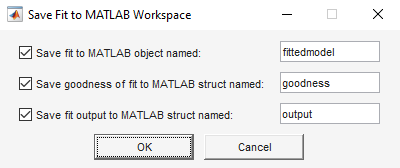## Generate Code and Export Fits to the Workspace

### Generating Code from the Curve Fitter App

You can generate and use MATLAB® code from an interactive session in the Curve Fitter app. In this way, you can transform your interactive analysis into reusable functions for batch processing of multiple data sets. You can use the generated file without modification, or you can edit and customize the file as needed.

To generate code for the currently selected fit and its opened plots in your Curve Fitter app session, follow these steps:

1. On the Curve Fitter tab, in the Export section, click and select Generate Code.

The Curve Fitter app generates code from your session and displays the file in the MATLAB Editor. The file includes the currently selected fit in your session and its opened plots. The file captures the following information:

• Name of the fit and its variables

• Fit settings and options

• Plots

• Curve or surface fitting object and the function used to create the fit:

• `cfit` or `sfit` object representing the fit

• A structure with goodness-of-fit information

2. Save the file.

To recreate your fit and its plots, call the file from the command line with your original data as input arguments. You also can call the file with new data.

For example, enter the following code, where `a`, `b`, and `c` are the variable names and `myFileName` is the file name.

`[fitresult,gof] = myFileName(a,b,c)`

Calling the file from the command line does not recreate your Curve Fitter app session. When you call the file, you get the same plots you had in your Curve Fitter app session in a standard MATLAB figure window. For example, if your fit in the Curve Fitter app session displayed fit, residuals, and contour plots, all three plots appear in a single figure window.

#### Curve Fitting Functions

The curve and surface fit objects (`cfit` and `sfit`) store the results from a fitting operation, making it easy to plot and analyze fits at the command line.

To learn about available functions for working with fits, see Curve and Surface Fitting.

### Exporting a Fit to the Workspace

To export a fit to the MATLAB workspace, follow these steps:

1. Select a fit and save it to the MATLAB workspace using one of these methods:

• Right-click the fit in the Table Of Fits pane, and select Save "`myfitname`" to Workspace.

• On the Curve Fitter tab, in the Export section, click and select Export to Workspace.

The app opens the Save Fit to MATLAB Workspace dialog box.2. Edit the names as appropriate. If you previously exported fits, the app automatically adds a numbered suffix to the default names to avoid overwriting existing names.

3. Choose which options you want to export by selecting the check boxes. Check box options are as follows:

• Save fit to MATLAB object named — This option creates a `cfit` or `sfit` object, that encapsulates the result of fitting a curve or surface to data. You can examine the fit coefficients at the command line.

`fittedmodel`
``` Linear model Poly22: fittedmodel(x,y) = p00 + p10*x + p01*y + p20*x^2 + p11*x*y + p02*y^2 where x is normalized by mean 1982 and std 868.6 and where y is normalized by mean 0.4972 and std 0.2897 Coefficients (with 95% confidence bounds): p00 = 0.4227 (0.3837, 0.4616) p10 = -0.137 (-0.1579, -0.1161) p01 = -0.1913 (-0.2122, -0.1705) p20 = 0.0234 (-2.895e-05, 0.04682) p11 = 0.067 (0.04613, 0.08787) p02 = -0.02946 (-0.05288, -0.006043)```
You also can treat the `cfit` or `sfit` object as a function to make predictions or evaluate the fit at values of X (or X and Y). See `cfit` and `sfit`.

• Save goodness of fit to MATLAB struct named — This option creates a structure that contains statistical information about the fit.

`goodness`
```goodness = struct with fields: sse: 9.4302 rsquare: 0.6565 dfe: 287 adjrsquare: 0.6505 rmse: 0.1813```

• Save fit output to MATLAB struct named — This option creates a structure that contains information such as number of observations, number of parameters, residuals, and so on.

`output`
```output = struct with fields: numobs: 293 numparam: 6 residuals: [293×1 double] Jacobian: [293×6 double] exitflag: 1 algorithm: 'QR factorization and solve' iterations: 1```

Note

The goodness-of-fit and output structures are outputs of the `fit` function.

4. Click to save the fit options to the workspace.

After you save your fit to the workspace, you can use fit postprocessing functions. For an example, see Analyzing Best Fit in the Workspace. For more information and a list of functions, see Fit Postprocessing.